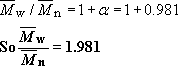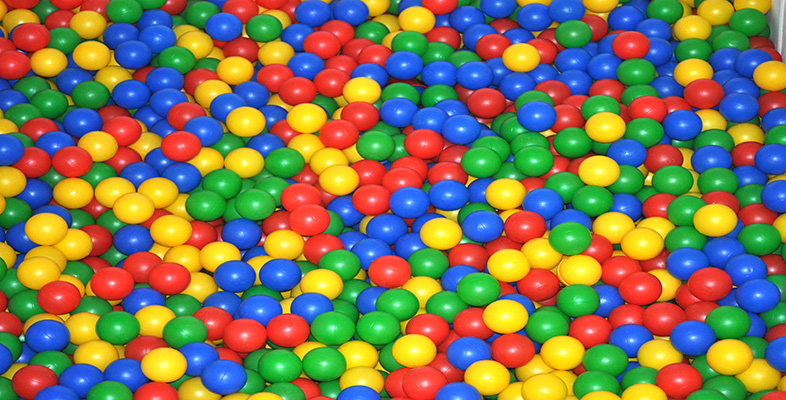Introduction to polymers

Start this free course now. Just create an account and sign in. Enrol and complete the course for a free statement of participation or digital badge if available.

Free course

# 4.4 Step growth polymerization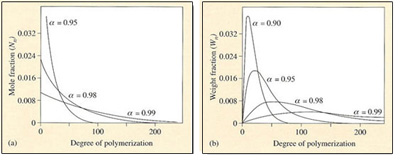Figure 41 Molecular mass distributions in step growth polymerization. The number distribution (a) is shown for three different extents of reaction, α. The mass distribution (b) shows a single narrow peak which progressively broadens and shifts to high molecular mass as α approaches unity

In contrast to chain growth reactions, where high molecular mass polymers are formed almost from the start of the reaction, a stepwise reaction results in the molecular mass of the polymer increasing slowly as the reaction progresses (Figure 41). Simple statistical arguments can be used to show how the distribution develops with extent of reaction. Sinceis simply the fraction of functional groups which has disappeared after time t (see Equation (13)), it can be interpreted as the probability that a functional group has reacted after time t. The probability of finding an unreacted functional group is thus (1 −). To determine the molecular mass distribution, it is necessary to find the probability that a molecule selected at random is an rimer. The probability of finding a single peptide group in a nylon molecule for example will be, and the probability of finding n − 1 of them will ben−1. The factor of unity appears because the end of the molecule will possess an unreacted carboxyl or amine group, which will have a probability of (1 −). So the probability of finding the complete n-mer is simplyn−1(1 −). This in turn is the fraction of n-mers in the entire assembly, so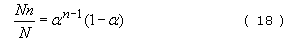where Nn is the number of n-mers present and N the total number of oligomers present. If the total number of repeat units present is N0, then N=N0(1 −). This is reasonable since when= 0, the total number of molecules present is just the number of monomer units present. As monomers combine together→ 1, N falls to a very small number and in the limit is just unity. Hence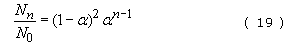This equation represents the number distribution function for a linear stepwise polymerization (Figure 41(a)).

The mass distribution is simply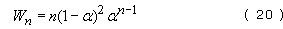and this distribution is shown in Figure 41(b) for several different extents of reaction. It can also be shown that the dispersion is given by the equation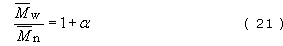so that as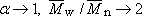.

High molecular mass material is developed only in the very final stages of reaction (= 0.99 and beyond). When the reaction is 90 per cent completed, the peak degree of polymerization is only about 10. For nylon 6, this represents a molecular mass of 1130. At 95 per cent completion, it is still only about 5650. Only at 99 per cent completion does the molecular mass begin to approach the useful region, with a peak of about 11 130. This feature of step-growth reactions creates severe problems for monomer purity (see Box 9). In fact the peak in the mass distribution is given by the equation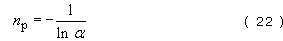and this value is close to the number-average molecular mass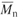. For thermoplastic polyesters, for example poly(ethylene terephthalate),must exceed about 10 000 for film formation and about 14 000 for fibre formation. For polyamides on the other hand,of commercial fibre forming polymer is 12 000–13 000, which for nylon 6,6 is equivalent to an average degree of polymerization of 53–58. The lower molecular masses needed for polyamides compared to polyesters reflects the strengthening characteristics of interchain hydrogen bonding.

## Self assessment question 7

An equi-unimolar mixture of terephthalic acid and ethylene glycol is polymerized to give PET. It is known that the glycol contains 1% by weight of methyl alcohol (CH3OH). What is the number-average molecular mass and dispersion of the PET so formed? The structures of acid and glycol are: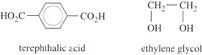The molecular mass of ethylene glycol = 24 + 32 + 6 = 62. Therefore the mixture contains 62 g of glycol of which 1 per cent or 0.62 g is methyl alcohol.

The molecular mass of methyl alcohol = 16 + 12 + 4 = 32. So the number of moles of CH3OH present = 0.62/32 = 0.0194 moles.

Now the hydroxyl (—OH) group in the alcohol will react with acidic groups to form dead polymer end groups: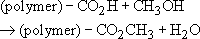The net effect will be to lower the molecular mass of the PET formed. The number of moles of glycol available for step growth polymerization will be 1 − 0.0194 = 0.9806. This then will be the maximum extent of reaction.

So= 0.9806

From Equation (22),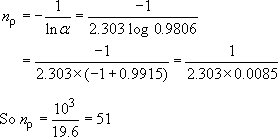The repeat unit molecular mass is 192 (Table 5), so that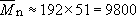From Equation (21)